# Subtracting Decimals

Let’s get straight to the point – learn how to subtract decimals with our easy guide.Author
Taylor HartleyExpert Reviewer

Published: August 24, 2023# Subtracting Decimals

Let’s get straight to the point – learn how to subtract decimals with our easy guide.Author
Taylor HartleyExpert Reviewer

Published: August 24, 2023# Subtracting Decimals

Let’s get straight to the point – learn how to subtract decimals with our easy guide.Author
Taylor HartleyExpert Reviewer

Published: August 24, 2023Key takeaways

• Adding and subtracting decimals is a lot easier than you think – You’ll use the same subtraction skills you use to subtract whole numbers.
• Understanding how decimals work makes everything simpler – Once you understand the different parts of a decimal number, it’s pretty easy to visualize adding and subtracting them.
• Practice makes perfect – The more time you take to practice your subtraction skills, the better you’ll be when it comes to subtracting decimals.

Has your mom, dad, or guardian ever given you an allowance? Maybe they’ve offered to pay you ten dollars to do your chores. When you write ten dollars out, it looks like this:

\$10.00

Now, if you stop doing your chores, your mom might threaten to decrease your allowance by \$2.50. That means you need to figure out how much you’re going to be making, given this new, frustrating arrangement (side note: do your chores!).

Subtracting decimals is an important skill. It will help you save your money, figure out how much money you can spend, and more. Let’s dive into how to subtract decimals.

## Adding and subtracting decimals, the rundownIt might look a little strange, but adding and subtracting decimals involves the same skills as finding the sum or the difference between two whole numbers.

First, always make sure that you put the number you start with on top and the number you are subtracting on the bottom.

Just like with normal addition and subtraction, you’ll want to arrange the equation vertically. This will make it easier to carry numbers and borrow numbers when you’re adding or subtracting.

Let’s see what that would look like.

You’re given two decimal numbers: 6.78 and 4.5

So you will arrange them with the larger number on the top and the smaller number on the bottom.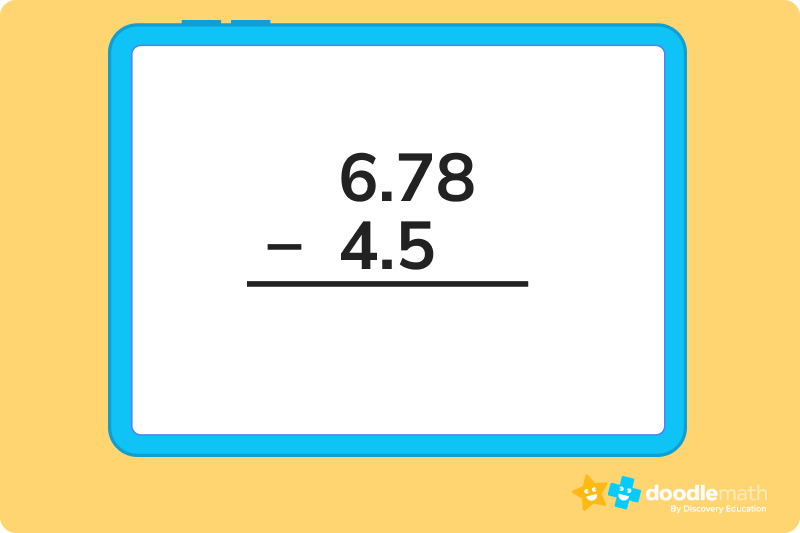Now, you’re probably noticing that these numbers don’t match up. There isn’t a number underneath the 8 in 6.78. You need the decimals to match – what do you do?

Add a zero to the end of 4.5 so that the number becomes 4.50. Now, your equation looks like this: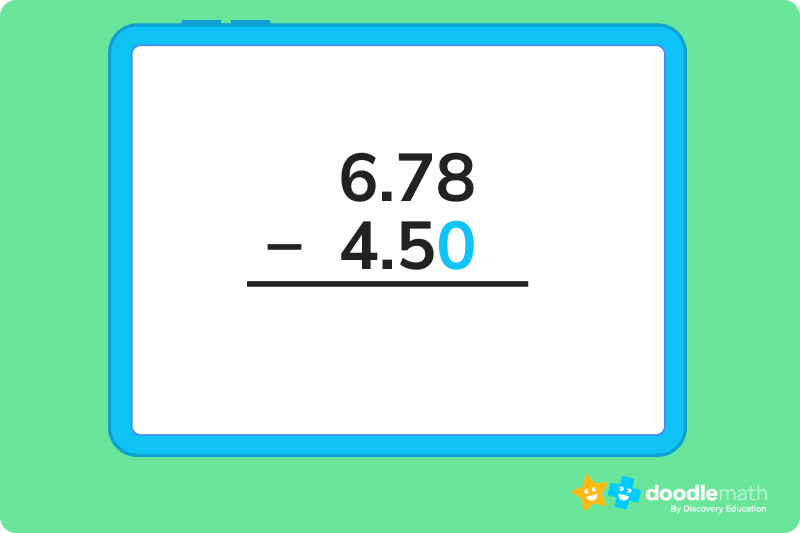## Important terms to knowIt always helps to break things down into smaller pieces in order to understand the big picture. Let’s break that funky-looking number down a little bit. There are three terms you need to know in order to understand decimals:

• Decimal number – This is the entire figure you’re working with, including the whole number and the fractional part
• Whole number – This is the number to the left of the decimal point, the whole number
• Fractional part – This is the number or numbers to the right of the decimal point, representing a part of a whole number

It might help if we visualize this together. Let’s take a look at decimal number 4.56.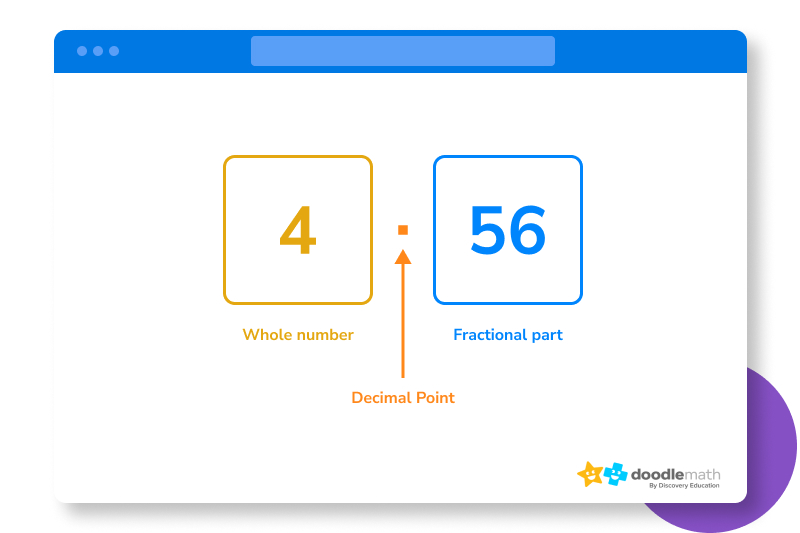## How to subtract decimals in 4 easy stepsSay we are given the following problem.

7.35 – 3.26

Let’s walk through a problem step-by-step to make sure we understand how to find the answer.

### 1. Arrange the problem verticallyMake sure you put the leading decimal number on top. It should look like this: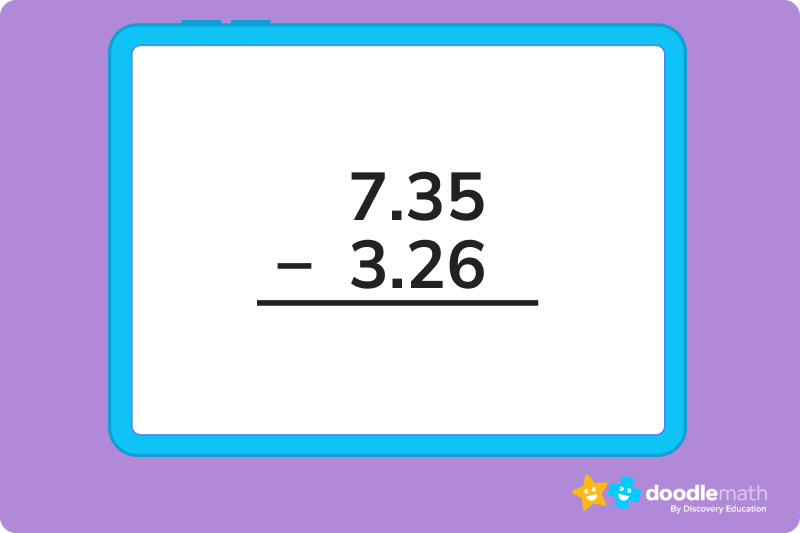### 2. Borrow where you need toKeep in mind that you can use place value to borrow numbers when you’re forced to subtract a larger number from a smaller one.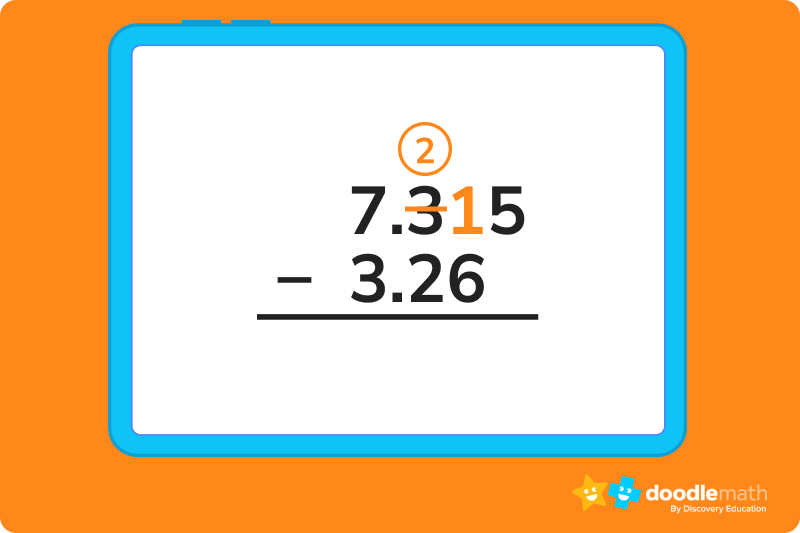### 3. SubtractNow that we’ve done our borrowing, we can start subtracting. It might be easiest for you to subtract one number from another at a time, then put the decimal number together at the end.

15 – 6 = 9. That means the second integer in the fractional number will be 9.

2 – 2 = 0. That means the first integer in the fractional number will be 0.

7 -3 = 4. So 4 is our whole number.

### 4. Put the decimal number togetherNow that we’ve done our subtraction, we should have our answer:

7.35 – 3.26 = 4.09

But don’t forget, sometimes, you have to add a zero to a fractional part to make sure you can subtract.

## Let’s practice together!Before we set you off on your own path, let’s do a few practice problems together. Each are asking you to subtract decimals, but they’re worded a little differently to test your knowledge. Let’s get started.

### 1. Miguel has \$12.56. He wants to buy a pack of gum for \$1.50. How much will Miguel have left if he buys the gum?Arrange the problem vertically first.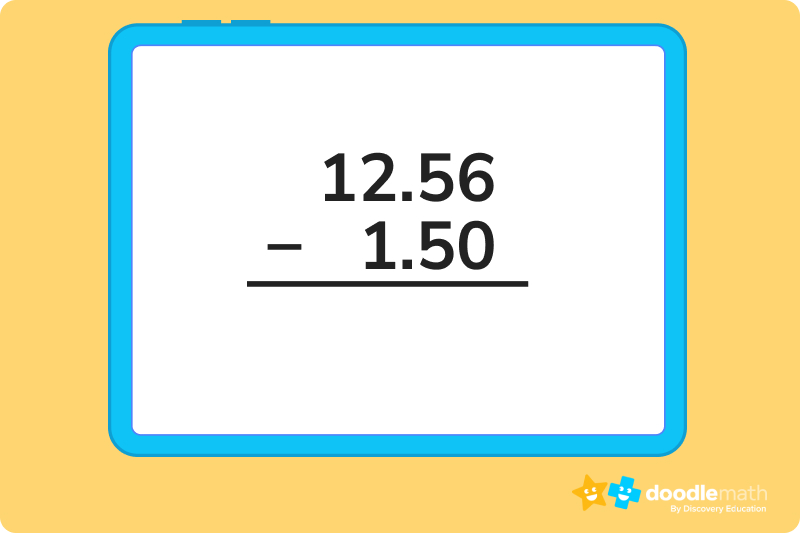Then, start subtracting, borrowing if and when you need to (here, we don’t have to borrow at all!)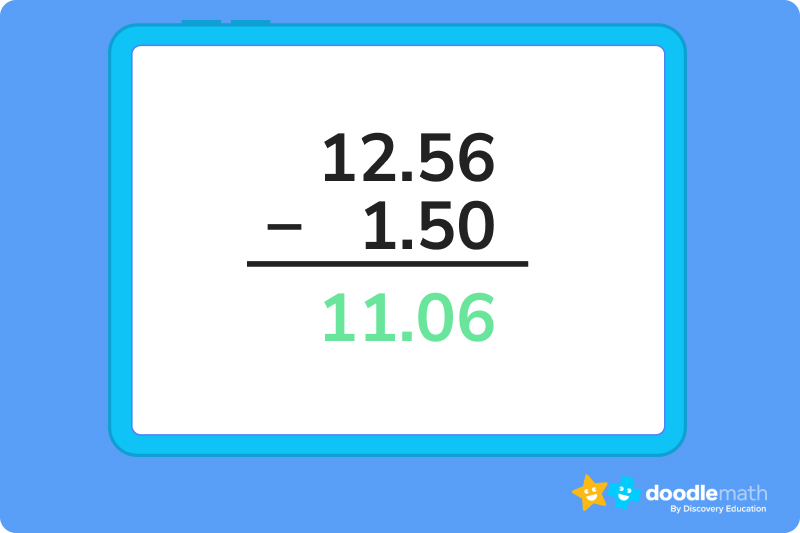### 2. Solve the following: 7.8 - 5.69.For this one, we’re going to have to make the decimals match up. Time to use our zeros!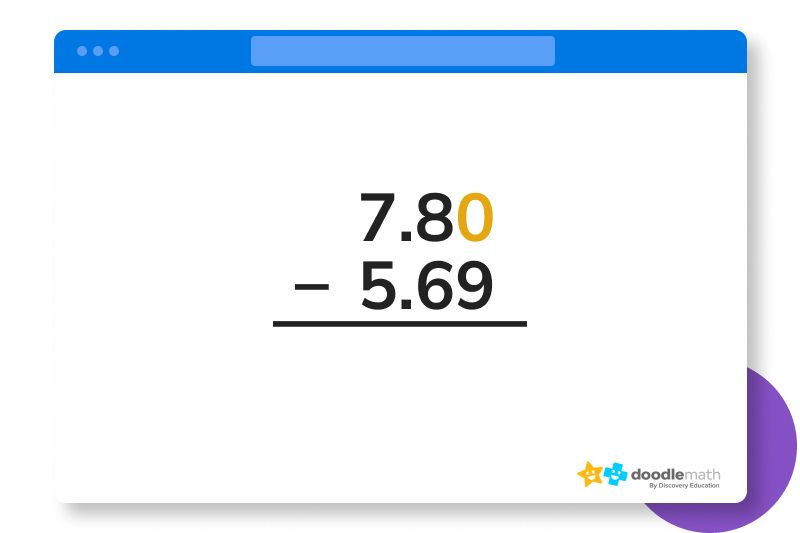Then, we need to borrow, because we can’t subtract 9 from 0. Once we do that, we can do our subtraction.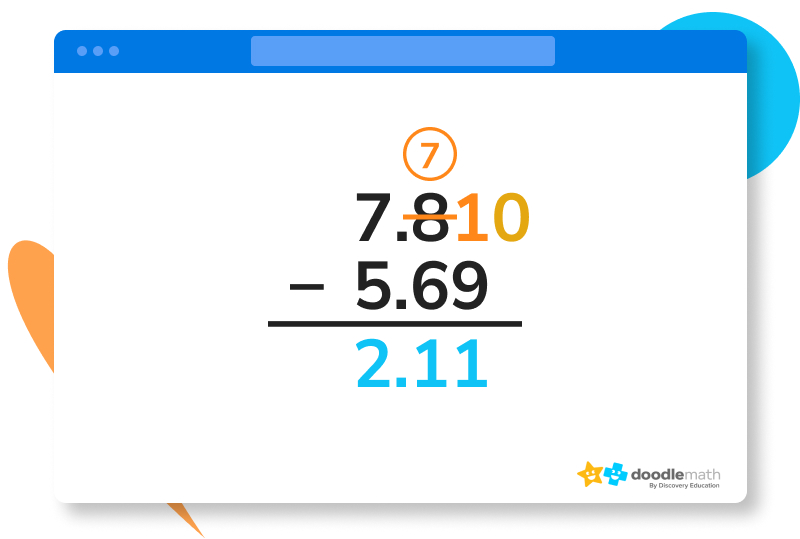### 3. Emily has an 8 hour shift. She has worked 4.5 hours. How many more hours does Emily have left to work?Once again, we have to use our zeros!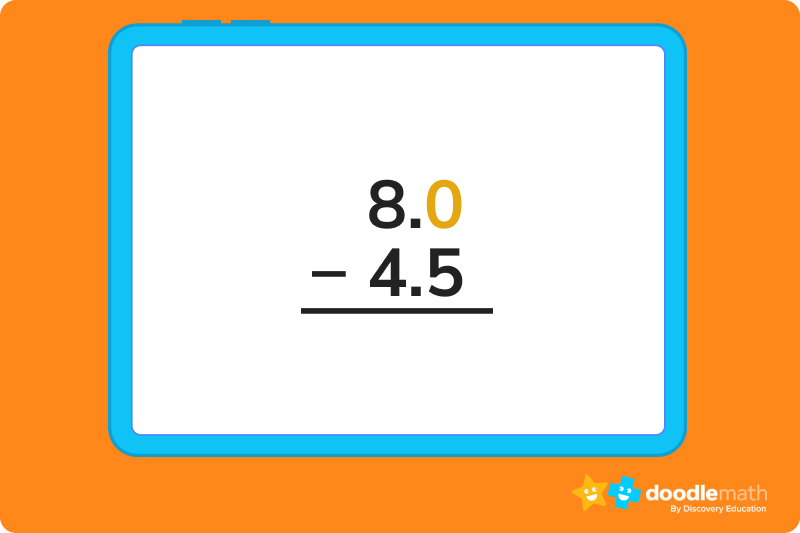Then, we have to borrow.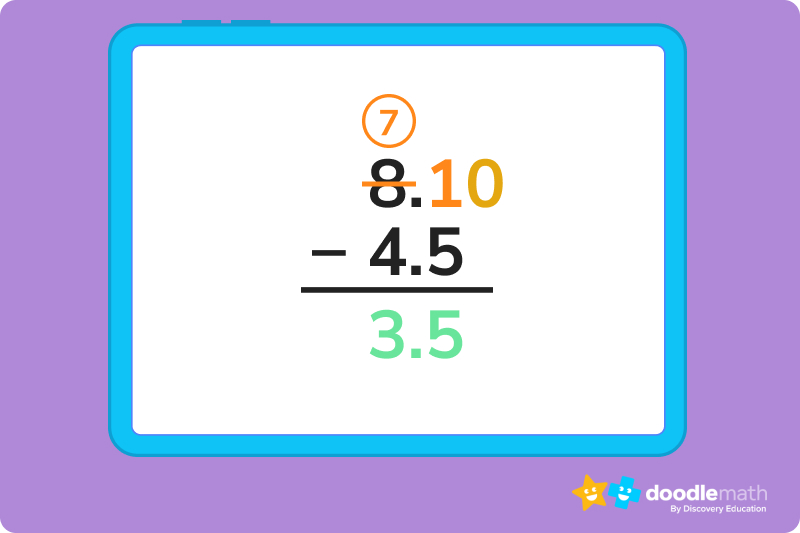Emily has 3.5 hours left on her shift.

Ready to give it a go?

Now it’s time for you to give a few of these problems a shot on your own. Feel free to consult the explanations, definitions, and practice problems above if you get confused or stumped on a question. And remember, practice makes perfect, so don’t get discouraged. Keep working through these problems and we promise you’ll get the hang of subtracting decimals in no time!

## Parent Guide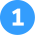How did we get here?

With this problem, you don’t have to borrow. Just have your student arrange the problem vertically, then subtract.

6.75

– 3.23

______

3.52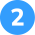How did we get here?

1. Arrange the numbers vertically.

25.00

-15.75

2. Borrow, borrow, borrow.

12145.910 0100

15.75

3. Go one number at a time starting with the whole number and subtract.

So 10 – 5 = 5

9 – 7 = 2

14 – 5 = 9

1 – 1 = 0

4. Put it all together, and you get \$9.25.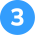How did we get here?

1. Arrange the problem vertically.

5.62

-2.71

2. Borrow where appropriate.

45.1662

-2.71

3. Subtract the numbers.

2 – 1 = 1

16 – 7 = 9

4 – 2 = 2

4. Put it all together, you get 2.91.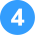How did we get here?

1. Arrange vertically.

11.5

– 4.25

11.50

– 4.25

3. Borrow where you need to.

11.45100

–    4.25

4. Subtract.

10 – 5 = 5

4 -2  = 2

11 – 4 = 7

5. Put it all together to get 7.25.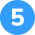How did we get here?

1. Arrange vertically.

6.98

-4.2

6.98

-4.20

3. Subtract.

6.98

-4.20

______

2.78

## FAQs about math strategies for kidsWe understand that diving into new information can sometimes be overwhelming, and questions often arise. That’s why we’ve meticulously crafted these FAQs, based on real questions from students and parents. We’ve got you covered!

There’s no single way to subtract decimals, but the first thing you want to do is line the numbers up vertically with the largest number on top and make sure the decimal points line up. Fill in any zeroes to make sure you have the same amount of numbers (so, for example, if you have 4.5 and 7.68, add a zero to 4.5 to make it 4.50). Then, subtract!

Decimals and fractions are simply two different ways to represent numbers. Fractions are written with the whole number in front and the fraction portion like A/B, with A representing part of the whole, B. Meanwhile, a decimal is written with the whole number to the left and the fractional part to the right.

So 4 ½ and 4.5 are the same number, written differently.

Yes you can! If you need to borrow in order to solve the problem, you can use that strategy to find the correct answer. It works the same way it does with double digit numbers that do not have decimals.## Related Posts

Title 1

Title 2

Title 3

Lesson credits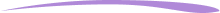Taylor Hartley

Taylor Hartley is an author and an English teacher based in Charlotte, North Carolina. When she's not writing, you can find her on the rowing machine or lost in a good novel.

Jill Padfield has 7 years of experience teaching high school mathematics, ranging from Alegra 1 to AP Calculas. She is currently working as a Business Analyst, working to improve services for Veterans while earning a masters degree in business administration.

Taylor Hartley

Taylor Hartley is an author and an English teacher based in Charlotte, North Carolina. When she's not writing, you can find her on the rowing machine or lost in a good novel.

Jill Padfield has 7 years of experience teaching high school mathematics, ranging from Alegra 1 to AP Calculas. She is currently working as a Business Analyst, working to improve services for Veterans while earning a masters degree in business administration.# Are you a parent, teacher or student?

Are you a parent or teacher?

## Hi there!

Book a chat with our team